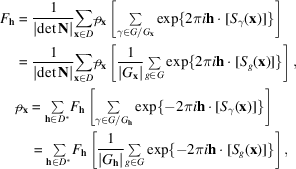International
Tables for
Crystallography
Volume B
Reciprocal space
Edited by U. Shmueli

International Tables for Crystallography (2010). Vol. B, ch. 1.3, pp. 77-78   | 1 | 2 |

## Section 1.3.4.3.2. Defining relations and symmetry considerations

G. Bricognea

aGlobal Phasing Ltd, Sheraton House, Suites 14–16, Castle Park, Cambridge CB3 0AX, England, and LURE, Bâtiment 209D, Université Paris-Sud, 91405 Orsay, France

#### 1.3.4.3.2. Defining relations and symmetry considerations

| top | pdf |

A finite set of reflectionscan be periodized without aliasing by the translations of a suitable sublatticeof the reciprocal lattice; the converse operation in real space is the sampling of ρ at points X of a grid of the form(Section 1.3.2.7.3). In standard coordinates,is periodized by, andis sampled at points.

In the absence of symmetry, the unique data are

 – theindexed byin reciprocal space; – theindexed by; or equivalently theindexed by, where.

In the presence of symmetry, the unique data areorin real space (by abuse of notation, D will denote an asymmetric unit for x or for m indifferently);in reciprocal space.

The previous summations may then be subjected to orbital decomposition, to yield the following crystallographic DFT' (CDFT) defining relations:with the obvious alternatives in terms of. Our problem is to evaluate the CDFT for a given space group as efficiently as possible, in spite of the fact that the group action has spoilt the simple tensor-product structure of the ordinary three-dimensional DFT (Section 1.3.3.3.1).

Two procedures are available to carry out the 3D summations involved as a succession of smaller summations:

 (1) decomposition into successive transforms of fewer dimensions but on the same number of points along these dimensions. This possibility depends on the reducibility of the space group, as defined in Section 1.3.4.2.2.4, and simply invokes the tensor product property of the DFT; (2) factorization of the transform into transforms of the same number of dimensions as the original one, but on fewer points along each dimension. This possibility depends on the arithmetic factorability of the decimation matrix N, as described in Section 1.3.3.3.2.

Clearly, a symmetry expansion to the largest fully reducible subgroup of the space group will give maximal decomposability, but will require computing more than the unique results from more than the unique data. Economy will follow from factoring the transforms in the subspaces within which the space group acts irreducibly.

For irreducible subspaces of dimension 1, the group action is readily incorporated into the factorization of the transform, as first shown by Ten Eyck (1973).

For irreducible subspaces of dimension 2 or 3, the ease of incorporation of symmetry into the factorization depends on the type of factorization method used. The multidimensional Cooley–Tukey method (Section 1.3.3.3.1) is rather complicated; the multidimensional Good method (Section 1.3.3.3.2.2) is somewhat simpler; and the Rader/Winograd factorization admits a generalization, based on the arithmetic of certain rings of algebraic integers, which accommodates 2D crystallographic symmetries in a most powerful and pleasing fashion.

At each stage of the calculation, it is necessary to keep track of the definition of the asymmetric unit and of the symmetry properties of the numbers being manipulated. This requirement applies not only to the initial data and to the final results, where these are familiar; but also to all the intermediate quantities produced by partial transforms (on subsets of factors, or subsets of dimensions, or both), where they are less familiar. Here, the general formalism of transposition (or orbit exchange') described in Section 1.3.4.2.2.2plays a central role.

### References

Ten Eyck, L. F. (1973). Crystallographic fast Fourier transforms. Acta Cryst. A29, 183–191.